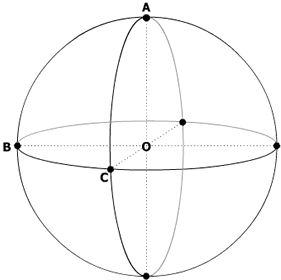#### You may also like### 2D-3D

Two circles of equal size intersect and the centre of each circle is on the circumference of the other. What is the area of the intersection? Now imagine that the diagram represents two spheres of equal volume with the centre of each sphere on the surface of the other. What is the volume of intersection?### The Dodecahedron Explained

What is the shortest distance through the middle of a dodecahedron between the centres of two opposite faces?### When the Angles of a Triangle Don't Add up to 180 Degrees

This article outlines the underlying axioms of spherical geometry giving a simple proof that the sum of the angles of a triangle on the surface of a unit sphere is equal to pi plus the area of the triangle.

# Mesh

##### Age 16 to 18Challenge LevelA wire frame is made by wrapping a wire around the 'equator' of a perfectly spherical balloon of unit radius and wrapping two wires at right angles around the sphere going through the North and South poles. The balloon is then deflated but remains perfectly spherical until it can just be moved out of the framework. What is the radius of the largest sphere that can pass through this frame, without distorting it, from inside to outside?